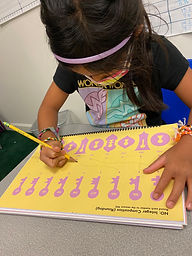## Ms. Brianne

### Target 1​

###### Lesson Type:

New

Number Operation

:

Integer Composition

Use place-value understandings to round whole numbers to the nearest ten or 100.

###### 1:

Use understanding of how to round two-digit numbers and expand the strategy to three-digit numbers.

###### 2:

Understand that rounding identifies the multiple of 10 or 100 that is closest to the given number.

###### 3:

Understand that the previous place (one place smaller) is the key digit to evaluate to determine whether a number is rounded up or down.

3rd

###### Vocabulary:

Rounding, Nearest Ten, Nearest Hundred

Activities:

• Students extended their knowledge of rounding to three-digit numbers.
• Students learned that they had to look at one place value smaller to determine whether to round up or down.
• Students rolled dice to generate a three-digit number and practiced rounding it to the nearest tens and ones.
• Students connected each number shown to its nearest 100.### Home Exploration

###### Guiding Questions:## Absent Students:

### Target 2

:

###### 1:

Understand that a graph is a visual representation of data.

###### 2:

Become familiar with the vocabulary associated with graphs: unit, scale, title and labels.

###### 3:

Construct and read a scaled bar graph.

3rd

###### Vocabulary:

Activities:

• Students used tally marks to collect data about a topic of their own choice.
• Students used their data to construct a bar graph.### Home Exploration

###### Guiding Questions:### Target 3

:

###### Vocabulary:

Activities:### Home Exploration